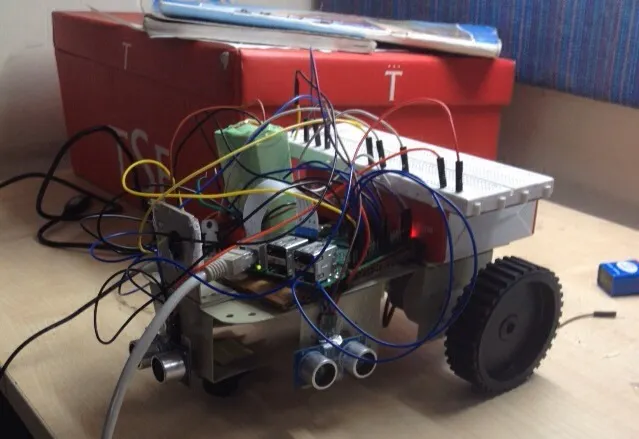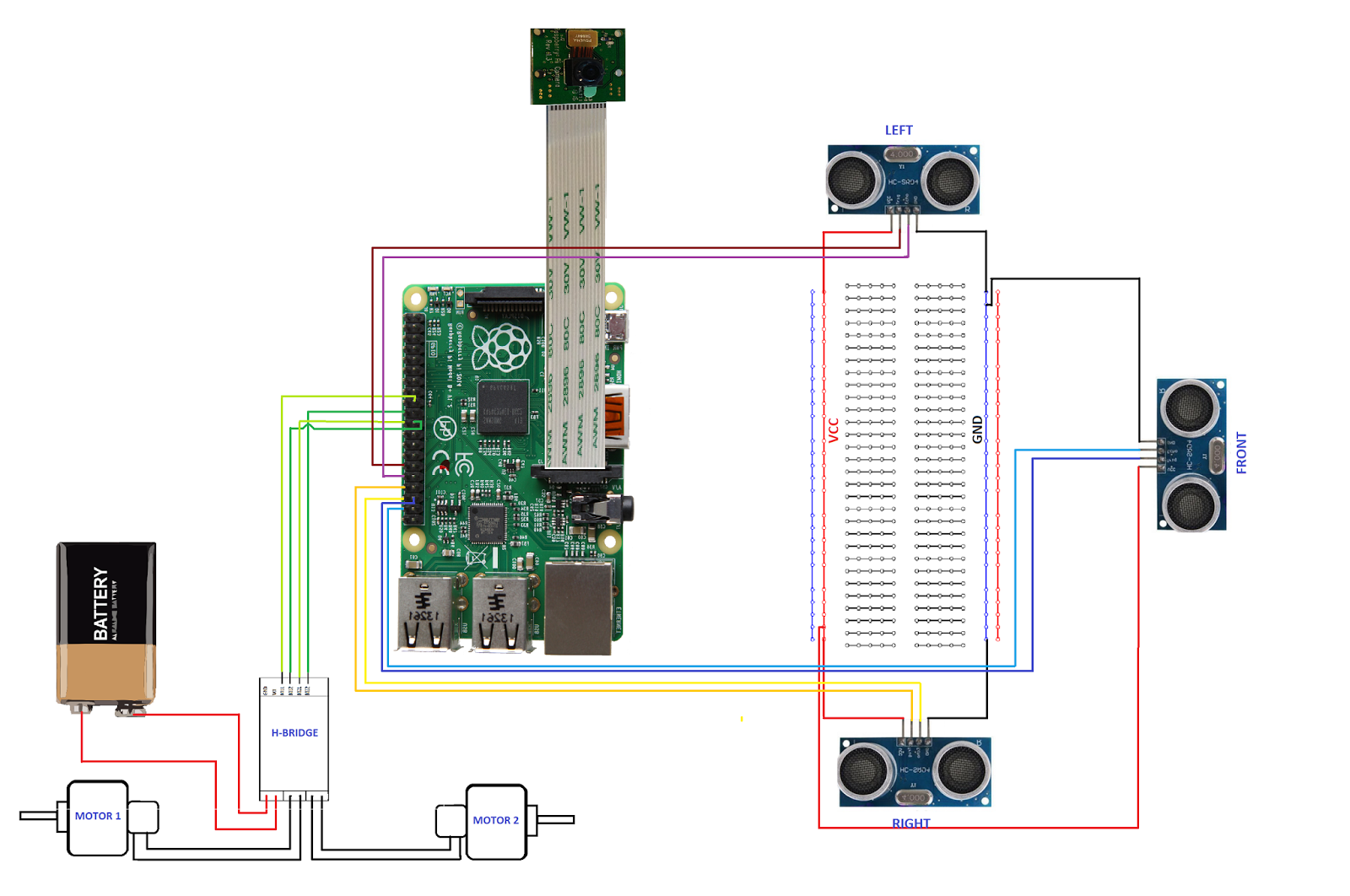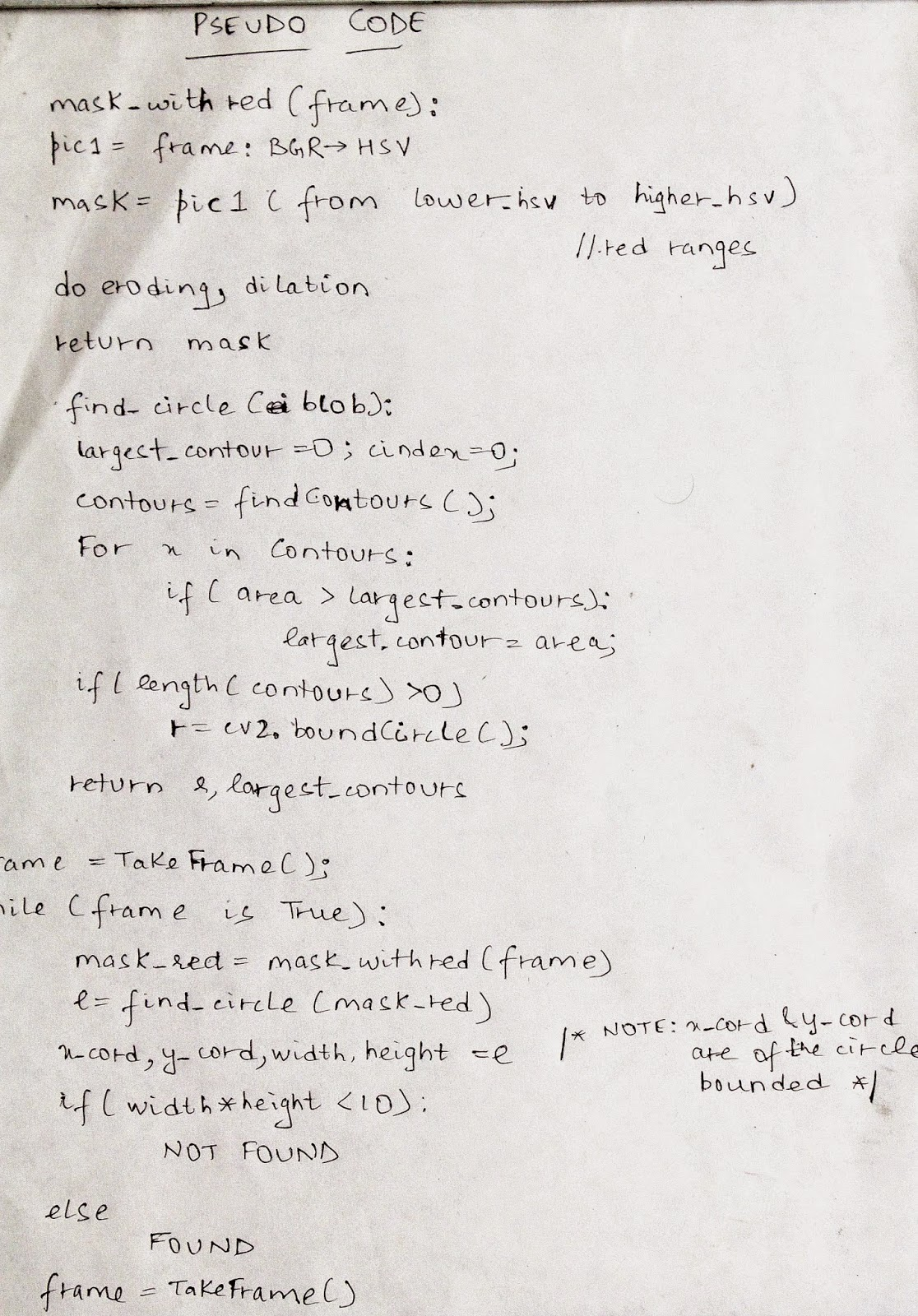Project showcaseBall Tracking Robot

A robo which follows an object of uniform color using Computer Vision.

• 70,196 views
• 116 respects

 Python

Apps and online services

The major drawback in today’s surveillance rests on the involvement of human operators which can easily be distracted, so we need a system which can autonomously monitor regions continuously, making decisions while identifying unwanted or obnoxious things and respond accordingly. Object tracking using computer vision is crucial in achieving automated surveillance.

I made this project in order to build a basic ball tracking car. Here, my bot uses camera to take frames and do image processing to track down the ball. The features of the ball such as color, shape, size can be used.

But my objective was to make a basic prototype for such a bot which can sense color and shape and follow it. My robot tries to find a color which is hard coded, if it finds a ball of that color it follows it.

I have chosen raspberry pi as micro-controller for this project as it gives great flexibility to use Raspberry Pi camera module and allows to code in Python which is very user friendly and OpenCV library, for image analysis.

For controlling the motors, I have used an H-Bridge to switch from clockwise to counter-clockwise or to stop the motors. This I have integrated via code when direction and speed has to be controlled in different obstacle situations.

Crucial thing while detecting images frame by frame was to avoid any frame drops as then the bot can go into a limbo state if the bot is unable to predict direction of ball after few frame drops. Even if it manage the frame drops then also if the ball goes out of scope of the camera, it will go into a limbo state, in that case, then I have made my bot take a 360 degree view of it's environment till the ball comes back in the scope of the camera and then start moving in it's direction.

For the image analysis, I am taking each frame and then masking it with the color needed. Then for noise reduction, I am eroding the noise and dilating the major blobs. Then I find all the contours and find the largest among them and bound it in a rectangle. And show the rectangle on the main image and find the coordinates of the center of the rectangle.I have attached the algorithm (pseudo-code) of the image analysis part and demonstrated this part in the video also.

Finally my bot tries to bring the coordinates of the ball to the center of its imaginary coordinate axis. This is how my robo works. I enjoy a lot working on this project and its a nice experience.

Video :

Code

CodePython
Upload in your Raspberry Pi and make a shell script to run it as soon as Raspberry Pi starts.
# import the necessary packages
from picamera.array import PiRGBArray     #As there is a resolution problem in raspberry pi, will not be able to capture frames by VideoCapture
from picamera import PiCamera
import RPi.GPIO as GPIO
import time
import cv2
import cv2.cv as cv
import numpy as np

#hardware work
GPIO.setmode(GPIO.BOARD)

GPIO_TRIGGER1 = 29      #Left ultrasonic sensor
GPIO_ECHO1 = 31

GPIO_TRIGGER2 = 36      #Front ultrasonic sensor
GPIO_ECHO2 = 37

GPIO_TRIGGER3 = 33      #Right ultrasonic sensor
GPIO_ECHO3 = 35

MOTOR1B=18  #Left Motor
MOTOR1E=22

MOTOR2B=21  #Right Motor
MOTOR2E=19

LED_PIN=13  #If it finds the ball, then it will light up the led

# Set pins as output and input
GPIO.setup(GPIO_TRIGGER1,GPIO.OUT)  # Trigger
GPIO.setup(GPIO_ECHO1,GPIO.IN)      # Echo
GPIO.setup(GPIO_TRIGGER2,GPIO.OUT)  # Trigger
GPIO.setup(GPIO_ECHO2,GPIO.IN)
GPIO.setup(GPIO_TRIGGER3,GPIO.OUT)  # Trigger
GPIO.setup(GPIO_ECHO3,GPIO.IN)
GPIO.setup(LED_PIN,GPIO.OUT)

# Set trigger to False (Low)
GPIO.output(GPIO_TRIGGER1, False)
GPIO.output(GPIO_TRIGGER2, False)
GPIO.output(GPIO_TRIGGER3, False)

# Allow module to settle
def sonar(GPIO_TRIGGER,GPIO_ECHO):
start=0
stop=0
# Set pins as output and input
GPIO.setup(GPIO_TRIGGER,GPIO.OUT)  # Trigger
GPIO.setup(GPIO_ECHO,GPIO.IN)      # Echo

# Set trigger to False (Low)
GPIO.output(GPIO_TRIGGER, False)

# Allow module to settle
time.sleep(0.01)

#while distance > 5:
#Send 10us pulse to trigger
GPIO.output(GPIO_TRIGGER, True)
time.sleep(0.00001)
GPIO.output(GPIO_TRIGGER, False)
begin = time.time()
while GPIO.input(GPIO_ECHO)==0 and time.time()<begin+0.05:
start = time.time()

while GPIO.input(GPIO_ECHO)==1 and time.time()<begin+0.1:
stop = time.time()

# Calculate pulse length
elapsed = stop-start
# Distance pulse travelled in that time is time
# multiplied by the speed of sound (cm/s)
distance = elapsed * 34000

# That was the distance there and back so halve the value
distance = distance / 2

print "Distance : %.1f" % distance
# Reset GPIO settings
return distance

GPIO.setup(MOTOR1B, GPIO.OUT)
GPIO.setup(MOTOR1E, GPIO.OUT)

GPIO.setup(MOTOR2B, GPIO.OUT)
GPIO.setup(MOTOR2E, GPIO.OUT)

def forward():
GPIO.output(MOTOR1B, GPIO.HIGH)
GPIO.output(MOTOR1E, GPIO.LOW)
GPIO.output(MOTOR2B, GPIO.HIGH)
GPIO.output(MOTOR2E, GPIO.LOW)

def reverse():
GPIO.output(MOTOR1B, GPIO.LOW)
GPIO.output(MOTOR1E, GPIO.HIGH)
GPIO.output(MOTOR2B, GPIO.LOW)
GPIO.output(MOTOR2E, GPIO.HIGH)

def rightturn():
GPIO.output(MOTOR1B,GPIO.LOW)
GPIO.output(MOTOR1E,GPIO.HIGH)
GPIO.output(MOTOR2B,GPIO.HIGH)
GPIO.output(MOTOR2E,GPIO.LOW)

def leftturn():
GPIO.output(MOTOR1B,GPIO.HIGH)
GPIO.output(MOTOR1E,GPIO.LOW)
GPIO.output(MOTOR2B,GPIO.LOW)
GPIO.output(MOTOR2E,GPIO.HIGH)

def stop():
GPIO.output(MOTOR1E,GPIO.LOW)
GPIO.output(MOTOR1B,GPIO.LOW)
GPIO.output(MOTOR2E,GPIO.LOW)
GPIO.output(MOTOR2B,GPIO.LOW)

#Image analysis work
def segment_colour(frame):    #returns only the red colors in the frame
hsv_roi =  cv2.cvtColor(frame, cv2.cv.CV_BGR2HSV)
mask_1 = cv2.inRange(hsv_roi, np.array([160, 160,10]), np.array([190,255,255]))
ycr_roi=cv2.cvtColor(frame,cv2.cv.CV_BGR2YCrCb)

kern_dilate = np.ones((8,8),np.uint8)
kern_erode  = np.ones((3,3),np.uint8)

def find_blob(blob): #returns the red colored circle
largest_contour=0
cont_index=0
contours, hierarchy = cv2.findContours(blob, cv2.RETR_CCOMP, cv2.CHAIN_APPROX_SIMPLE)
for idx, contour in enumerate(contours):
area=cv2.contourArea(contour)
if (area >largest_contour) :
largest_contour=area

cont_index=idx
#if res>15 and res<18:
#    cont_index=idx

r=(0,0,2,2)
if len(contours) > 0:
r = cv2.boundingRect(contours[cont_index])

return r,largest_contour

def target_hist(frame):
hsv_img=cv2.cvtColor(frame, cv2.COLOR_BGR2HSV)

hist=cv2.calcHist([hsv_img],,None,,[0,255])
return hist

#CAMERA CAPTURE
#initialize the camera and grab a reference to the raw camera capture
camera = PiCamera()
camera.resolution = (160, 120)
camera.framerate = 16
rawCapture = PiRGBArray(camera, size=(160, 120))

# allow the camera to warmup
time.sleep(0.001)

# capture frames from the camera
for image in camera.capture_continuous(rawCapture, format="bgr", use_video_port=True):
#grab the raw NumPy array representing the image, then initialize the timestamp and occupied/unoccupied text
frame = image.array
frame=cv2.flip(frame,1)
global centre_x
global centre_y
centre_x=0.
centre_y=0.
hsv1 = cv2.cvtColor(frame, cv2.COLOR_BGR2HSV)
x,y,w,h=loct

#distance coming from front ultrasonic sensor
distanceC = sonar(GPIO_TRIGGER2,GPIO_ECHO2)
#distance coming from right ultrasonic sensor
distanceR = sonar(GPIO_TRIGGER3,GPIO_ECHO3)
#distance coming from left ultrasonic sensor
distanceL = sonar(GPIO_TRIGGER1,GPIO_ECHO1)

if (w*h) < 10:
found=0
else:
found=1
simg2 = cv2.rectangle(frame, (x,y), (x+w,y+h), 255,2)
centre_x=x+((w)/2)
centre_y=y+((h)/2)
cv2.circle(frame,(int(centre_x),int(centre_y)),3,(0,110,255),-1)
centre_x-=80
centre_y=6--centre_y
print centre_x,centre_y
initial=400
flag=0
GPIO.output(LED_PIN,GPIO.LOW)
if(found==0):
#if the ball is not found and the last time it sees ball in which direction, it will start to rotate in that direction
if flag==0:
rightturn()
time.sleep(0.05)
else:
leftturn()
time.sleep(0.05)
stop()
time.sleep(0.0125)

elif(found==1):
if(area<initial):
if(distanceC<10):
#if ball is too far but it detects something in front of it,then it avoid it and reaches the ball.
if distanceR>=8:
rightturn()
time.sleep(0.00625)
stop()
time.sleep(0.0125)
forward()
time.sleep(0.00625)
stop()
time.sleep(0.0125)
#while found==0:
leftturn()
time.sleep(0.00625)
elif distanceL>=8:
leftturn()
time.sleep(0.00625)
stop()
time.sleep(0.0125)
forward()
time.sleep(0.00625)
stop()
time.sleep(0.0125)
rightturn()
time.sleep(0.00625)
stop()
time.sleep(0.0125)
else:
stop()
time.sleep(0.01)
else:
#otherwise it move forward
forward()
time.sleep(0.00625)
elif(area>=initial):
initial2=6700
if(area<initial2):
if(distanceC>10):
#it brings coordinates of ball to center of camera's imaginary axis.
if(centre_x<=-20 or centre_x>=20):
if(centre_x<0):
flag=0
rightturn()
time.sleep(0.025)
elif(centre_x>0):
flag=1
leftturn()
time.sleep(0.025)
forward()
time.sleep(0.00003125)
stop()
time.sleep(0.00625)
else:
stop()
time.sleep(0.01)

else:
#if it founds the ball and it is too close it lights up the led.
GPIO.output(LED_PIN,GPIO.HIGH)
time.sleep(0.1)
stop()
time.sleep(0.1)
#cv2.imshow("draw",frame)
rawCapture.truncate(0)  # clear the stream in preparation for the next frame

if(cv2.waitKey(1) & 0xff == ord('q')):
break

GPIO.cleanup() #free all the GPIO pins

SchematicsSmart Face Tracking Robot Car

Project tutorial by Sebastian Hernandez

• 14,868 views
• 53 respects

MeArm Robot Arm - Your Robot - V1.0

Project tutorial by Benjamin Gray

• 43,110 views
• 58 respects

Omni-Directional People Tracking Friendly Robot

Project tutorial by Think Engineer

• 9,702 views
• 32 respects

Elliot the Line Follower Robot

Project showcase by SMM2

• 15,939 views
• 28 respects

• 89,264 views